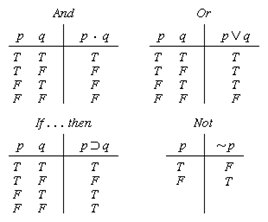# Setting Up Truth Tables Worksheets

How to Create a Truth Table In a Boolean Algebra, the expression involves bools or true or false values. The true and false are represented as T and F, respectively. The value of T is 1, and the value of F is 0.to create a truth table, we need to understand Boolean algebra. The Boolean algebra depends on binary digits (0,1). We take two events into consideration. It is an easy way to express difficult and lengthy statements into easy to comprehend logical expressions. There are various operators involved in creating a truth table, Such as; AND, OR, NOR, XOR. Let's see how to use these logical operators. The AND operator (symbolically: ∧), also known as logical conjunction requires both p and q to be True for the result to be True. The OR operator (symbolically: ∨) requires only one premise to be True for the result to be True. Logical NOR (symbolically: ↓) is the exact opposite of OR. It requires both p and q to be False to result in True. Exclusive Or, or XOR for short, (symbolically: ⊻) requires exactly one True and one False value in order to result in True.

• ### Basic Lesson

Demonstrates the concept of setting up truth tables. Set up a truth table to identify the possible truth values of a single, simple statement P. 1. A single statement such as P has two possible truth values. It can be either true or false. 2. The truth table will need three rows: one for the statement, one for each possible truth values. 3. Write the statement (P) on the top left cell (left of the double line) and list the possible truth values below it (T, F).

• ### Intermediate Lesson

Shows students how to to setup and solve truth values. The truth table will need five rows: one for the statements and one for each possible combination: a. both T b. the first T and the second F c. the first F and the second T d. both F

• ### Independent Practice 1

Contains a mixture of problems that ask students to setup truth tables. Set up truth tables to identify the possible truth values of the following single, simple statements.

• ### Independent Practice 2

Features setting up truth value questions with assorted concepts.

• ### Homework Worksheet

Features 6 truth table creation problems.

• ### Skill Quiz

10 truth value questions that require setup. Scoring matrix is provided.

• ### Homework and Quiz Answer Key

Answers for the homework and quiz.

Answers for the lesson and practice sheets.

• ### Basic Lesson

Demonstrates the concept of determining assorted and, or, if...then (implies) skills for truth values.

• ### Intermediate Lesson

Shows students how to determine assorted truth values.

• ### Independent Practice 1

Contains a mixture of problems using assorted truth values.

• ### Independent Practice 2

Features truth value questions with assorted concepts.

• ### Homework Worksheet

Features 6 assorted truth-value problems.

• ### Skill Quiz

10 assorted truth value questions. Scoring matrix is provided.

• ### Homework and Quiz Answer Key

Answers for the homework and quiz.